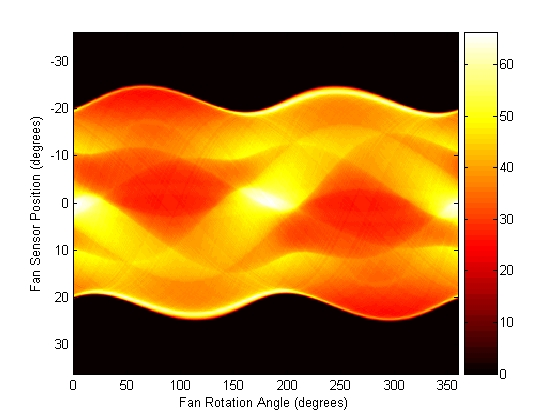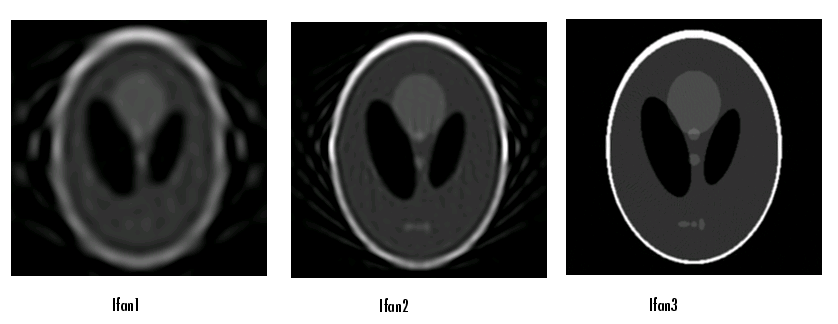Image Processing Toolbox User's GuideWorking with Fan-Beam Projection Data

The commands below illustrate how to use `fanbeam` and `ifanbeam` to form projections from a sample image and then reconstruct the image from the projections. The test image is the Shepp-Logan head phantom, which can be generated by the Image Processing Toolbox function `phantom`. The phantom image illustrates many of the qualities that are found in real-world tomographic imaging of human heads.

1. Generate the test image and display it.
• ```P = phantom(256);
imshow(P)```
2. Compute fan-beam projection data of the test image, using the `FanSensorSpacing` parameter to vary the sensor spacing. The example uses the `fanbeam` arc geometry, so you specify the spacing between sensors by specifying the angular spacing of the beams. The first call spaces the beams at 2 degrees; the second at 1 degree; and the third at 0.25 degrees. In each call, the distance between the center of rotation and vertex of the projections is constant at 250 pixels. In addition, `fanbeam` rotates the projection around the center pixel at 1 degree increments.
• ```D = 250;

dsensor1 = 2;
F1 = fanbeam(P,D,'FanSensorSpacing',dsensor1);

dsensor2 = 1;
F2 = fanbeam(P,D,'FanSensorSpacing',dsensor2);

dsensor3 = 0.25
[F3, sensor_pos3, fan_rot_angles3] = fanbeam(P,D,...
'FanSensorSpacing',dsensor3);
```
3. Plot the projection data `F3`. Because `fanbeam` calculates projection data at rotation angles from 0 to 360 degrees, the same patterns occur at an offset of 180 degrees. The same features are being sampled from both sides. Compare this plot to the plot of the parallel-beam projection data of the head phantom on page 8-33.
• ```figure, imagesc(fan_rot_angles3, sensor_pos3, F3)
colormap(hot); colorbar
xlabel('Fan Rotation Angle (degrees)')
ylabel('Fan Sensor Position (degrees)')```
4. Reconstruct the image from the fan-beam projection data using `ifanbeam`. In each reconstruction, match the fan sensor spacing with the spacing used when the projection data was created in step 2. The example uses the `OutputSize` parameter to constrain the output size of each reconstruction to be the same as the size of the original image `|P|`.
• ```output_size = max(size(P));

Ifan1 = ifanbeam(F1,D,
'FanSensorSpacing',dsensor1,'OutputSize',output_size);
figure, imshow(Ifan1)

Ifan2 = ifanbeam(F2,D,
'FanSensorSpacing',dsensor2,'OutputSize',output_size);
figure, imshow(Ifan2)

Ifan3 = ifanbeam(F3,D,
'FanSensorSpacing',dsensor3,'OutputSize',output_size);
figure, imshow(Ifan3)
```
1. The following figure shows the result of each transform. Note how the quality of the reconstruction gets better as the number of beams in the projection increases. The first image, `Ifan1`, was created using 2 degree spacing of the beams; the second image, `ifan2`, was created using 1 degree spacing of the beams; the third image, `ifan3`, was created using 0.25 spacing of the beams.Reconstructions of the Head Phantom Image from Fan-Beam ProjectionsReconstructing an Image from Fan-Beam Projection Data Morphological Operations